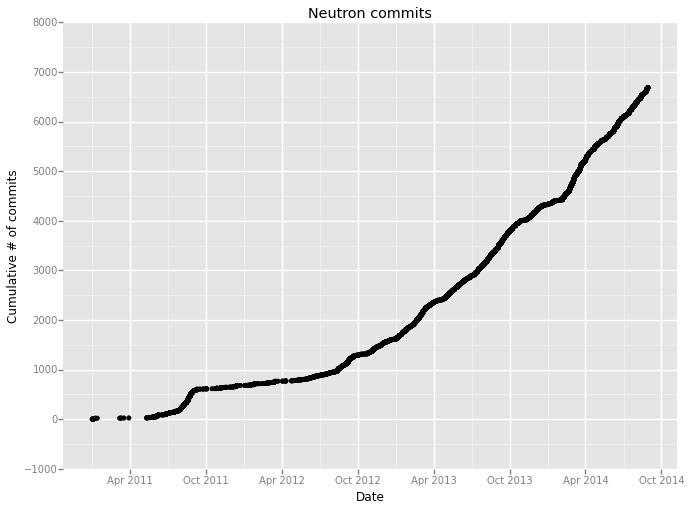### Plotting counting processes with IPython, Pandas and ggplot¶

In :
import pandas as pd


Generate the commit dates from the OpenStack Neutron project in reverse chronological order:

$git clone https://github.com/openstack/neutron.git$ cd neutron
\$ git log --pretty=format:'%cd' > commits.txt
In :
df_raw = pd.read_table('commits.txt', names=["Date"])

In :
# The dates aren't strictly reverse chronological order for earlier
# commits for some reason, so we just sort them here
# We keep them in reverse order to demonstrate flipping the data later
dates = sorted(pd.to_datetime(df_raw["Date"]), reverse=True)

In :
# Create a time series where the indexes are chronological,
# and the values are just 1
# Here we do the flip with the ::-1 indexing
ts = pd.Series(1, index=dates[::-1])

In :
# Create a data frame with the cumulative
df = pd.DataFrame(ts.cumsum(), columns=["Count"])
# We want "Date" to be a column instead of the index so we can plot it
# See http://stackoverflow.com/a/24374962/742
dt = df.index
df['Date'] = dt
df = df.reset_index(drop=True)

In :
from ggplot import *
%matplotlib inline
p = ggplot(aes(x='Date',y='Count'), data=df) + \
geom_point() + ylab("Cumulative # of commits") + \
ggtitle("Neutron commits")

In :
# Show it inline
pOut:
<ggplot: (274395169)>
In :
# Save it to a file
ggsave(p, "cumsum.png")

Saving 11.0 x 8.0 in image.

In :